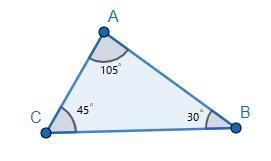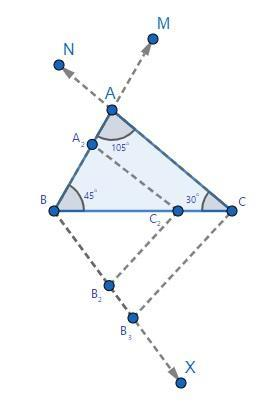Courses
Courses for Kids
Free study material
Free LIVE classes
MoreLIVE
Join Vedantu’s FREE Mastercalss

# Draw a triangle $ABC$ with side $BC=6cm,\text{ }\angle C={{30}^{\circ }}$ and $\angle A={{105}^{\circ }}$. Then construct another triangle whose sides are $\dfrac{2}{3}$ times the corresponding sides of $\Delta ABC$.Verified
363.6k+ views
Hint: We have two of the angles known in a triangle, then using the sum of the angle property of a triangle we can easily calculate the third angle. It will help in determining the triangle’s rough structure. Further, the exact triangle can be drawn with the help of known side length.

Here, we have $\angle C={{30}^{\circ }}$ and $\angle A={{105}^{\circ }}$ of a triangle $ABC$, and from the sum angle property of a triangle, we have
$\Rightarrow \angle A+\angle B+\angle C={{180}^{\circ }}...\text{ }\left( 1 \right)$
Substituting the values of $\angle A$ and $\angle C$ in equation (1), we get
\begin{align} & \Rightarrow \angle A+\angle B+\angle C={{180}^{\circ }} \\ & \Rightarrow {{105}^{\circ }}+\angle B+{{30}^{\circ }}={{180}^{\circ }} \\ & \Rightarrow \angle B+{{135}^{\circ }}={{180}^{\circ }} \\ \end{align}
On transposing values in the above equation, we get
\begin{align} & \Rightarrow \angle B+{{135}^{\circ }}={{180}^{\circ }} \\ & \Rightarrow \angle B={{180}^{\circ }}-{{135}^{\circ }} \\ & \Rightarrow \angle B={{45}^{\circ }} \\ \end{align}
A rough structure of the triangle can be known, i.e.,
Now, to build up the exact structure of a given triangle, we have to use constructions, i.e., there would be certain steps of construction to build exact angles with exact side lengths.
Thus, steps of construction are:Now, to build up the exact structure of a given triangle, we have to use constructions, i.e., there would be certain steps of construction to build exact angles with exact side lengths.
Thus, steps of construction are:

1.Drawing a line $BC$ of length $6cm$.
2.Then, drawing a ray $CN$ making an angle of ${{30}^{\circ }}$ at vertex $C$.
3.Again, drawing a ray $BM$ making an angle of ${{45}^{\circ }}$ at vertex $B$.
4.Now, there would be a point of intersection of rays $CN$ and $BM$ which would definitely be vertex $A$.
5.This $ABC$ is the triangle we build with given dimensions but we have to draw a similar triangle with sides of length $\dfrac{2}{3}$of corresponding length of sides of $\Delta ABC$.
6.Thus, drawing a ray $BX$ making an acute angle with $BC$ on the side opposite to the vertex $A$.
7.Now, dividing the whole length of $BX$ into three equal parts and naming them as ${{B}_{1}},{{B}_{2}},{{B}_{3}}$ along the length towards $X$, i.e., $B{{B}_{1}}={{B}_{1}}{{B}_{2}}={{B}_{2}}{{B}_{3}}$.
8.Now, to create a complete triangle join ${{B}_{3}}C$ and drawing a line parallel to ${{B}_{3}}C$ from point ${{B}_{2}}$ towards the line $BC$, intersecting it at point let’s say, ${{C}_{2}}$.
9.Again, drawing a line from point ${{C}_{2}}$ towards line $AB$ parallel to $AC$, intersecting it at point ${{A}_{2}}$.
10.Thus, a new triangle formed on joining these lines, we get $\Delta {{A}_{2}}B{{C}_{2}}$ as our required triangle with corresponding sides equal to $\dfrac{2}{3}$ length of $\Delta ABC$.Hence, $\Delta {{A}_{2}}B{{C}_{2}}$ is our required triangle with corresponding sides equal to $\dfrac{2}{3}$ length of $\Delta ABC$.

Note: There are several ways to construct similar triangles, having sides’ lengths correspondingly equal to other triangles. But an easier way could be to use the similar triangle properties, to find the exact length of sides of the new triangle and then construct it, using AAA or AA properties.

Last updated date: 01st Oct 2023
Total views: 363.6k
Views today: 11.63k